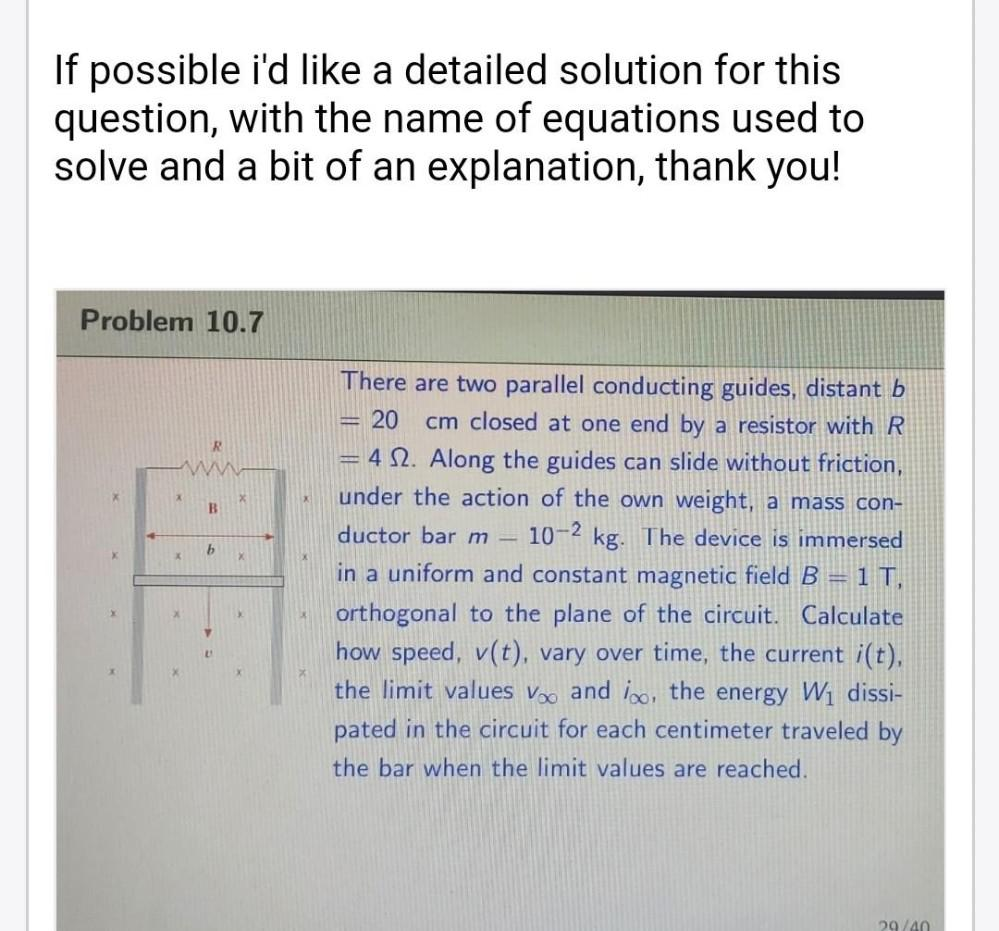Question:

# If possible i'd like a detailed solution for this question, with the name of equations used to solve and a bit of an explanationIf possible i'd like a detailed solution for this question, with the name of equations used to solve and a bit of an explanation, thank you! Problem 10.7 R W x B b X There are two parallel conducting guides, distant b 20 cm closed at one end by a resistor with R = 4 12. Along the guides can slide without friction, under the action of the own weight, a mass con- ductor barm 10-2 kg. The device is immersed in a uniform and constant magnetic field B=1 T, orthogonal to the plane of the circuit. Calculate how speed, v(t), vary over time, the current i(t), the limit values Voo and iso, the energy W1 dissi- pated in the circuit for each centimeter traveled by the bar when the limit values are reached. 29/40